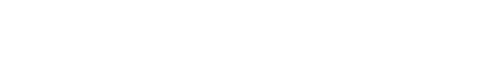# Proof-of-Loss

(Since January 03, 2018, the paper includes the pseudocode for block validation.)

An alternative consensus algorithm to both proof-of-work and proof-of-stake, proof-of-loss addresses all their deficiencies, including the lack of an organic block size limit, the risks of mining centralization, and the “nothing at stake” problem:

https://proof-of-loss.money/

1 Like

So what is the verdict on this? Those are some pretty big claims.

1 Like

How does proof-of-loss address the “nothing at stake” problem?

1. Transferring money requires transaction rights, which cost fees.
2. Each transaction consumes hence loses exactly as much in rights as its total size in bytes.
3. The spendable outputs of each transaction represent that loss.
4. The odds of chaining a block depend on the loss each of those outputs represents.
5. The reward for chaining each block is transaction rights instead of their price (in fees).
6. This reward is only saleable (for fees) in subsequent blocks.
7. If the same spendable output tries to chain two different blocks at the same height, then its earned rights are inherited by the spendable output chaining a child of either block.

This algorithm forces people to serially rather than parallelly chain blocks (“forcibly serial chaining” in the paper).

There was an error on page 5 of the paper, which made the block-chaining odds formula confusing. The error was in the text, not in the formula, and consisted on assuming the affected route as always being the rewarded one, which is false. The corrected version is already available at the same URL. The new file’s date is 02/07/2017, and downloading it will probably require clearing the browser’s cache.

In addition to corrections and improvements, the paper has now an introduction with examples:

https://proof-of-loss.money/

The paper now includes the pseudocode for block validation:

https://proof-of-loss.money/